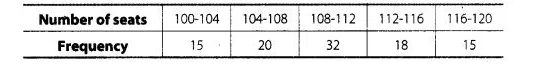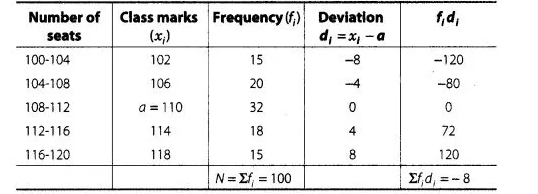# An aircraft has 120 passenger seats.

Question:

An aircraft has 120 passenger seats. The number of seats occupied during 100 flights is given in the following table.Determine the mean number of seats occupied over the flights.

Solution:

We first, find the class mark xi, of each class and then proceed as follows.$\therefore \quad$ Assumed mean, $a=110$,

Class width, $h=4$

and total observation, $N=100$

By assumed mean method,

$\operatorname{Mean}(\bar{x})=a+\frac{\Sigma f_{j} d_{j}}{\Sigma f_{i}}$

$=110+\left(\frac{-8}{100}\right)=110-0.08=109.92$